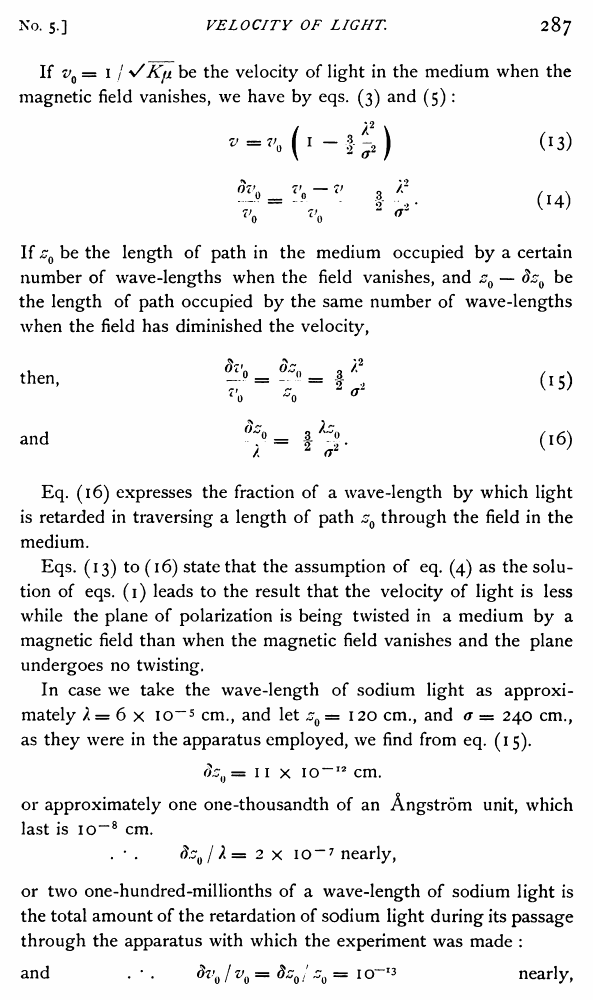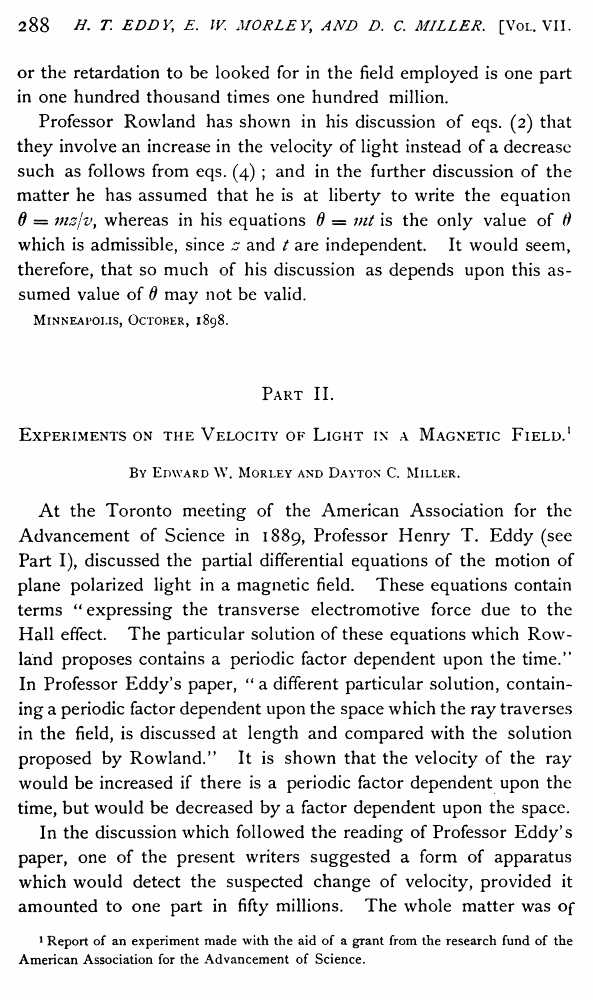Henry T. Eddy, Edward W. Morley, Dayton C. Miller. The Velocity of Light in The Magnetic Field //Physical Review (Series I), Volume 7, Issue 5 (1898)

В начало   Другие форматы   <<<     Страница 287   >>>283  284  285  286  287 288  289  290  291  292  293  294  295  296

If v0 = I / VKji be the velocity of light in the medium when the magnetic field vanishes, we have by eqs. (3) and (5):

VmtVo (! -f^2) (13)

t£- (14)

If z0 be the length of path in the medium occupied by a certain number of wave-lengths when the field vanishes, and z0 — dz0 be the length of path occupied by the same number of wave-lengths when the field has diminished the velocity,

then, - 0 = = I- (15)

70 ~0 ~ °

and °"° = (16)

/ z <jz v '

Eq. (16) expresses the fraction of a wave-length by which light is retarded in traversing a length of path s0 through the field in the medium.

Eqs. (13) to (16) state that the assumption of eq. (4) as the solution of eqs. (1) leads to the result that the velocity of light is less while the plane of polarization is being twisted in a medium by a magnetic field than when the magnetic field vanishes and the plane undergoes no twisting.

In case we take the wave-length of sodium light as approximately / = 6 x io-5 cm., and let zQ = 120 cm., and a — 240 cm., as they were in the apparatus employed, we find from eq. (15).

(hi} = 11 x io~12 cm.

or approximately one one-thousandth of an Angstrom unit, which last is io“8 cm.

. • . dz0/ ?,= 2 x io-7 nearly,

or two one-hundred-millionths of a wave-length of sodium light is the total amount of the retardation of sodium light during its passage through the apparatus with which the experiment was made :

and . * . <J?'0 / v0 = dz0/ z0 = io~13 nearly,

or the retardation to be looked for in the field employed is one part in one hundred thousand times one hundred million.

Professor Rowland has shown in his discussion of eqs. (2) that they involve an increase in the velocity of light instead of a decrease such as follows from eqs. (4) ; and in the further discussion of the matter he has assumed that he is at liberty to write the equation 0 = mz/v, whereas in his equations 6 = mt is the only value of 6 which is admissible, since z and t are independent. It would seem, therefore, that so much of his discussion as depends upon this assumed value of d may not be valid.

Minneapolis, October, 1898.

Part II.

Experiments on the Velocity of Light in a Magnetic Field.1

By Edward W. Morley and Dayton C. Miller.

At the Toronto meeting of the American Association for the Advancement of Science in 1889, Professor Henry T. Eddy (see Part I), discussed the partial differential equations of the motion of plane polarized light in a magnetic field. These equations contain terms “ expressing the transverse electromotive force due to the Hall effect. The particular solution of these equations which Rowland proposes contains a periodic factor dependent upon the time/’ In Professor Eddy’s paper, “ a different particular solution, containing a periodic factor dependent upon the space which the ray traverses in the field, is discussed at length and compared with the solution proposed by Rowland.” It is shown that the velocity of the ray would be increased if there is a periodic factor dependent upon the time, but would be decreased by a factor dependent upon the space.

In the discussion which followed the reading of Professor Eddy’s paper, one of the present writers suggested a form of apparatus which would detect the suspected change of velocity, provided it amounted to one part in fifty millions. The whole matter was of

1 Report of an experiment made with the aid of a grant from the research fund of the American Association for the Advancement of Science.